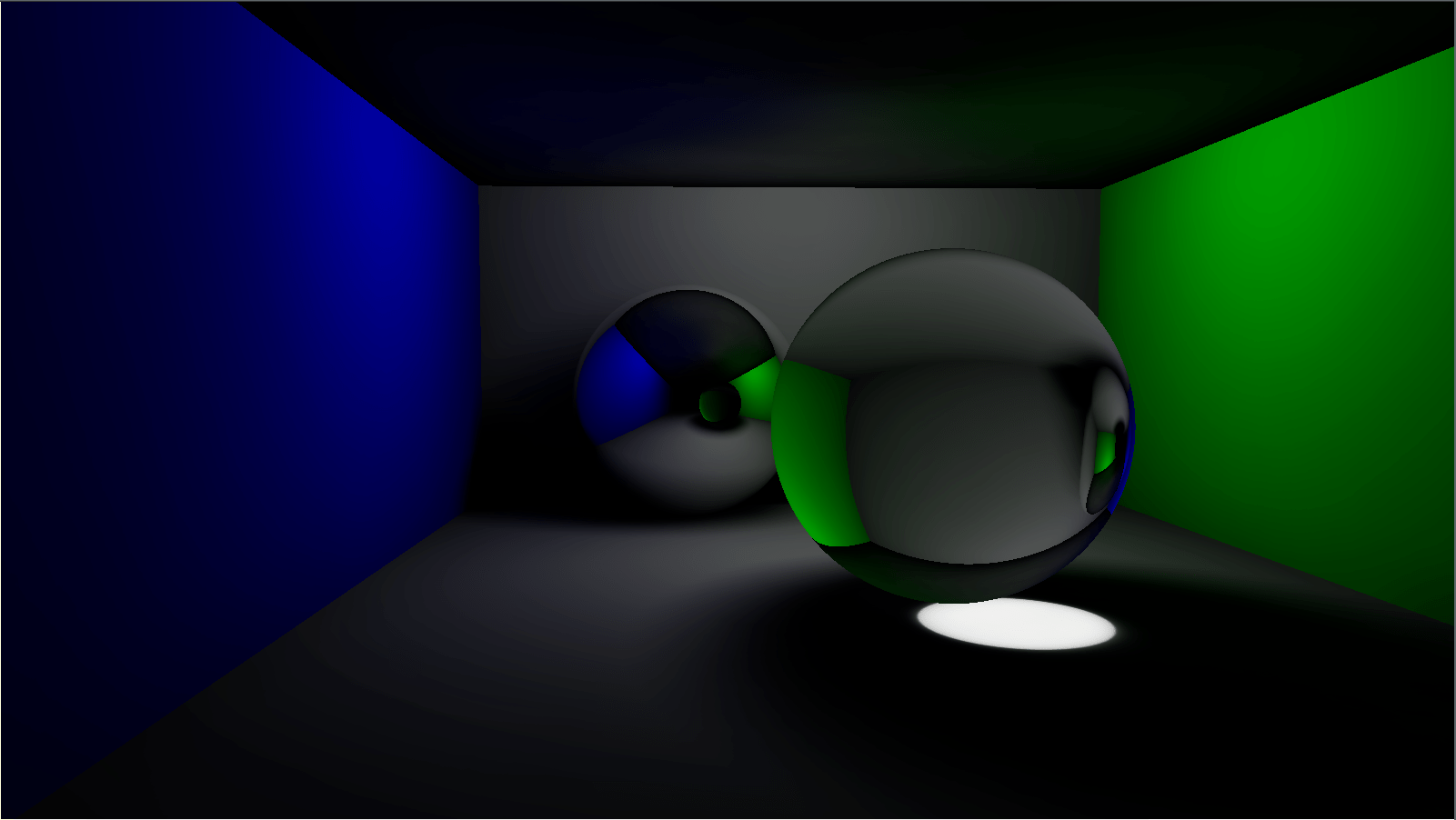# How to make a Photon Mapper : Photons everywhereBleed Color and Caustics.

Hello,

It has been a long time since I posted anything on this blog, I am so sorry about it.
I will try to diversify my blog, I’ll talk about C++, Rendering (always <3), and Qt, a framework I love.

So, in this last part, we will talk about photons.

## What exactly are Photons ?

A photon is a quantum of light. It carries the light straightforwardly on the medium. Thanks to it, we can see objects etc.

## How could we transform photons in a “visible value” like RGB color ?

We saw that the eyes only “see” the radiance !
So we have to transform our photons in radiance.

### From physic of photons

We know that one photon have for energy :$\displaystyle{}E_{\lambda}={h\nu}=\frac{hc}{\lambda}$

where$\lambda$ is the wavelength in nm and$E$ in Joules.
Say we have$n_\lambda$ photons of$E_{\lambda}$ each.
We can lay the luminous energy right now :$\displaystyle{Q_{\lambda}=n_{\lambda}E{\lambda}}$

The luminous flux is just the temporal derivative about the luminous energy :$\displaystyle{\phi_{\lambda}=\frac{dQ_{\lambda}}{dt}}$

The idea is great, but we have a luminous flux function of the wavelength, but the radiance is waiting for a general luminous flux
So, we want to have a general flux which is the integral over all wavelengths in the visible spectrum of the$\lambda$ luminous flux.$\displaystyle{\phi=\int_{380}^{750}d\phi_{\lambda}=\int_{380}^{750}\frac{\partial \phi_{\lambda}}{\partial\lambda}d\lambda}$

Now, we have the radiance$\displaystyle{L=\frac{d^2 \phi}{cos \theta dAd\omega}=\frac{d^2(\int_{380}^{750}\frac{\partial \phi_{\lambda}}{\partial \lambda}d\lambda)}{cos(\theta)dAd\omega}=\int_{380}^{750}\frac{d^3\phi_{\lambda}}{cos(\theta)dAd\omega d\lambda}d\lambda}$

Using the rendering equation, we get two forms :$\displaystyle{L^O=\int_{380}^{750}\int_{\Omega^+}fr(\mathbf{x}, \omega_i,\omega_o,\lambda)\frac{d^3\phi_{\lambda}}{dAd\lambda}d\lambda}$$\displaystyle{\int_{\Omega^+}fr(\mathbf{x}, \omega_i,\omega_o)\frac{d^2\phi}{dA}}$

The first one take care about dispersion since the second doesn’t.
In this post, I am not going to use the first one, but I could write an article about it latter.

## Let’s make our Photon Mapper

### What do we need ?

We need a Light which emits photons, so we could add a function “emitPhotons” .

We also need a material which bounces photons :

Obviously, we also need a structure for our photons. This structure should be able to store photons and compute irradiance at a given position.

### How could we do this ?

Photon emitting is really easy :

We divide the total flux by the number of photons and we compute a random direction, then we could trace the photon

Bouncing a photon depends on your material :

To take care about conservation of energy, we play Russian roulette.
Obviously, to take care about conservation of energy, we have to modify the direct lighting as well ^^.

Finally, we need to compute the irradiance at a given position :
It is only :$\displaystyle{E=\sum \frac {\phi}{\pi r^2}}$

So we could easily write :

To have shadows, you could emit shadow photons like this :

That’s all ! If you have any question, please, let me know !